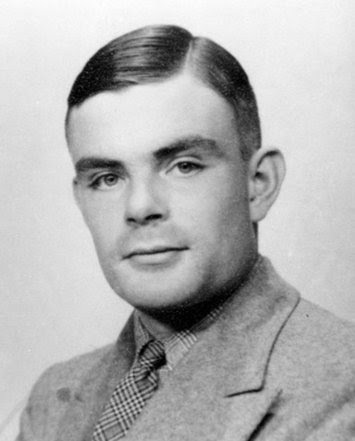### The entropy and the halting probability problem

The third law of thermodynamics states:
It is impossible for any procedure to lead to the isotherm $T = 0$ in a finite number of steps.
The theorem, discovered by Walther Nernst, is equal to say:
It is impossible for any process, no matter how idealized, to reduce the entropy of a system to its zero point value in a finite number of operations.
In classical thermodynamics we can define entropy, or the variation of entropy $\Delta S$, with the following equation: $\Delta S = \frac{\Delta Q}{T}$ where $\Delta Q$ is the heat's variation and $T$ is the temperature.
In 1970s Ludwig Boltzmann developed the statistical mechanics definition of the entropy, well known like Boltzmann's equation: $S = - k_B \sum_i p_i \ln p_i$ where $k_B$ is the Boltzmann's constant, $p_i$ is the probability that the system is in the $i$-th microstate.
Now we jump to 2007 when Gregory Chaitin defined the halting probability problem: $\Omega = \sum_{\{ p \}} 2^{-p} = \sum_i 2^{-p_i}$ $\Omega_i = 2^{-p_i}$ $p_i = - \lg_2 \Omega_i$ $p = \sum_i p_i = - \sum_i \lg_2 \Omega_i$The halting problem is the need to determine, given an arbitrary computer program, whether it will ever finish its processing or go on forever (something not too different from Hilbert's tenth problem, in some ways).
Alan Turing, in 1936, proved that there is no algorithm capable of solving the halting problem for all possible programs. Fundamental to this proof is the Turing machine itself: within this context, taking up the work of Kurt Godel, the problem of stopping is undecidable.
Chaitin then defined a halting probability(1), a real number which basically represents the probability that a randomly generated program will stop. This number turns out to be normal and transcendental, which implies that it can be well defined but cannot be fully calculated.
This means that it can be used to prove that there are no algorithms that produce the digits of $\Omega$, although its first digits have been calculated for simple cases, for example in binary notation: $\Omega = .110110\cdots$
1. G. J. Chaitin (2006). The Halting Probability Omega: Irreducible Complexity in Pure Mathematics Enriques lecture arXiv: math/0611740v1
Chaitin, G. (2006). The Limits of Reason Scientific American, 294 (3), 74-81 DOI: 10.1038/scientificamerican0306-74
Calude, C., & Stay, M. (2006). Natural halting probabilities, partial randomness, and zeta functions Information and Computation, 204 (11), 1718-1739 DOI: 10.1016/j.ic.2006.07.003
↩︎

#### 1 comment:

1.Thank you Mr. Turing.

Markup Key:
- <b>bold</b> = bold
- <i>italic</i> = italic
- <a href="http://www.fieldofscience.com/">FoS</a> = FoS